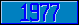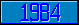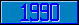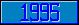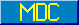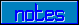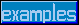## \$%MTXINV

### M[UMPS] by Example

```
2-Jul–96, 6:54:35

Routine Save for Matrix Mathematics Library function \$%MTXINV^MATH

;

; Unless otherwise noted, the code below

; was approved in document X11/96–26

;

; If corrections have been applied,

; first the original line appears,

; with three semicolons at the beginning of the line.

;

; Then the source of the correction is acknowledged,

; then the corrected line appears, followed by a

; line containing three semicolons.

;

MTXINV(A,R,N) ;

; Invert A[N,N], result goes to R[N,N]

IF \$DATA(A)<10 QUIT 0

IF \$GET(N)<1 QUIT 0

;

NEW T,X

SET X=\$%MTXUNIT^MATH(.T,N)

QUIT \$%MTXEQU^MATH(.A,.T,.R,N,N)

;===

;

;

;

```